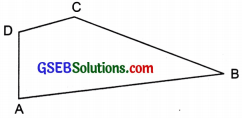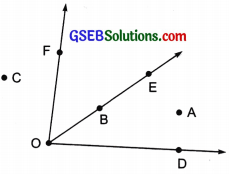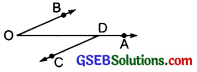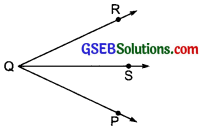# GSEB Solutions Class 6 Maths Chapter 4 Basic Geometrical Ideas Ex 4.3

Gujarat Board GSEB Textbook Solutions Class 6 Maths Chapter 4 Basic Geometrical Ideas Ex 4.3 Textbook Questions and Answers.

## Gujarat Board Textbook Solutions Class 6 Maths Chapter 4 Basic Geometrical Ideas Ex 4.3

Question 1.
Name the angles in the given figure.Solution:
The angles are:Question 2.
In the given diagram, name the point (s)
(a) In the interior of $$\angle DOE$$
(b) In the exterior of $$\angle EOF$$
(c) On $$\angle EOF$$Solution:
(a) Point in the interior of $$\angle DOE$$ is A.
(b) Points in the exterior of $$\angle EOF$$ are A, C and D.
(c) Points on $$\angle EOF$$ are : E, B, O and F.Question 3.
Draw rough diagrams of two angles such that they have
(a) One point in common.
(b) Two points in common.
(c) Three points in common.
(d) Four points in common.
(e) One ray in common.
Solution:
(a) In the figure below, $$\angle PQS$$ and $$\angle RQS$$ have one point Q in common.(b) In the figure below, $$\angle DOB$$ and $$\angle ODC$$ have two points O and D in common.(c) Not possible
(d) Not possible
(e) In the figure below, $$\angle RQS$$ and $$\angle PQS$$ have one ray $$\overline{\mathrm{QS}}$$ in common.